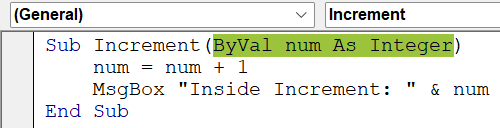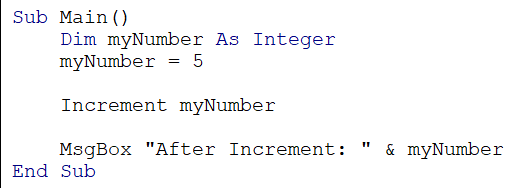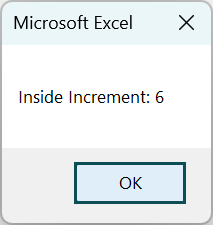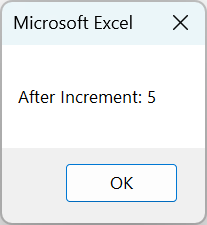# VBA ByValArticle byExcelMojo Team## What is Excel VBA ByVal Function Argument?

VBA ByVal (short form for “By Value”) is a function argument declaration used to pass values to a procedure or function. When a parameter is declared with ByVal, the argument’s value is passed to the procedure or function, making a copy of it. It means that any changes made to the parameter within the procedure or function do not affect the original value of the argument.

• Here’s a small example that demonstrates the usage of ByVal in VBA. The “MultiplyByTwo” subroutine takes an argument “number,” declared as an Integer, and passed by value using ByVal.
• Inside the subroutine, the value of “number” is multiplied by 2.
• In the “Main” subroutine, a variable “myNumber” is declared and assigned the value 5.
• The “MultiplyByTwo” subroutine is called with “myNumber” as an argument. Since ByVal is used, a copy of “myNumber” is passed to the “MultiplyByTwo” subroutine.
• After the subroutine call, a message box is displayed, showing the modified value of the “number.”

When the second message box is displayed, it shows the original value of “myNumber” from the “Main” subroutine because the modification made inside the “MultiplyByTwo” subroutine using ByVal, does not affect the original value of “myNumber,” which is the number “5.”

###### Key Takeaways
• ByVal is a function argument declaration in VBA that passes a copy of the value to a procedure or function without affecting the original value.
• Using ByVal is helpful when you want to prevent modifications to the original values of the arguments.
• VBA ByVal Default provides a default value for a parameter if no specific value is provided when calling a subroutine or function.
• When passing objects using ByVal, modifications to the object’s properties can still affect the original object outside the procedure or function.

### How to Use ByVal Argument in VBA?

To use the VBA ByVal argument, follow these steps.

1. Define a procedure or function that requires arguments. For example:In the “Increment” subroutine, an argument “num” is declared an Integer and passed by value using ByVal. Inside the subroutine, the value of “num” is incremented by 1, and a message box is displayed, showing the updated value of “num.”

2. Call the procedure or function and pass an argument by value. For example:In the “Main” subroutine, a variable “myNumber” is declared and assigned 5. The “Increment” subroutine is called with “myNumber” as an argument. Since ByVal is used, a copy of “myNumber” is passed to the “Increment” subroutine. After the subroutine call, another message box is displayed, showing the original value of “myNumber.”

3. Now, when you run the code, you will find that the first message box appears, which says “inside Increment: 6.”After you click OK, another message box says, “After Increment: 5.”The purpose of this code is to illustrate the behavior of ByVal in VBA.

Code:

Sub Increment (ByVal num As Integer)
num = num + 1
MsgBox “Inside Increment:” & num
End Sub

Sub Main ()
Dim myNumber As Integer
myNumber = 5
Increment myNumber
MsgBox “After Increment: ” & myNumber
End Sub

Explanation:

When you pass the “myNumber” variable to the “Increment” subroutine using ByVal, any changes made to “num” within the subroutine will not impact the original value of “myNumber” in the “Main” subroutine. The modifications are confined to the subroutine’s local copy of the variable.

It showcases the concept of passing arguments by value, where changes made within a subroutine do not persist outside of it.

### Examples

Let us look at some examples depicting the usage of ByVal in VBA.

#### Example #1

Let’s use VBA ByVal to calculate a Number’s Square in this example.

• Step 1: Open the VBA Editor by pressing Alt + F11 in Excel.
• Step 2: Insert a new module by clicking “Insert” from the top menu and selecting “Module.”
• Step 3: Define the Square subroutine that takes an argument number declared with ByVal.
• We define a subroutine called Square, which takes an argument number declared as a Double and passes by value using ByVal.
• Inside the subroutine, we declare a variable result of type Double.
• We calculate the square of the number by multiplying it by itself and assign the result to the result.
• A message box is displayed, showing the square of the number using the result variable.
• Step 4: Define the Main subroutine.
• We define a subroutine called “Main().”
• We declare a variable “inputNumber” of type Double.
• The “InputBox” function is used to prompts to enter a number, and the value entered by the user is assigned to “inputNumber.”
• The Square subroutine is called with “inputNumber” as an argument.
• Step 5: A dialog box appears when the VBA code is run, prompting the user to enter a number.
• Step 6: After entering the number, the Square subroutine is called with the entered number as an argument. For example, let us enter the number 27.
• Step 7: The Square subroutine calculates the square of the number and displays it in a message box. The output shows the square of the input number, which is 729.

Here is the full code:

Sub Square(ByVal number As Double)
Dim result As Double
result = number * number
MsgBox “The square of ” & number & ” is: ” & result
End Sub

Sub Main()
Dim inputNumber As Double
inputNumber = InputBox(“Enter a number:”)
Square inputNumber
End Sub

#### Example #2

In this example we will illustrate how ByVal allows us to pass arguments by value, ensuring that modifications made within a subroutine do not persist outside of it.

• Step 1: Define the ModifyString subroutine that takes an argument text declared with ByVal.
• We define a subroutine called ModifyString, which takes an argument text declared as a String and passes by value using VBA ByVal.
• Inside the subroutine, we modify the value of ‘text’ by concatenating “Modified: ” with its original value.
• A message box shows the modified value of ‘text.
• Step 2: Define the Main subroutine.
• We define a subroutine called “Main.”
• We declare a variable message of VBA data type String and assign it “Hello.”
• The ModifyString subroutine is called “message” as an argument.
• Since VBA ByVal is used, a copy of the “message” is passed to the ModifyString subroutine.
• After the subroutine call, a message box is displayed, showing the original value of the “message.”
• Step 3: When the code is run, the ModifyString subroutine is called with the message variable as an argument.

Inside the subroutine, the value of the text is modified by concatenating “Modified: ” with the original value of the text.

A message box is displayed, showing the modified value of text inside the subroutine.

• Step 4: After the subroutine call, another message box is displayed, showing the original value of the message.

The output demonstrates that the modification made to text inside the ModifyString subroutine does not affect the original value of the message due to the use of VBA ByVal.

Here is the full code:

Sub ModifyString(ByVal text As String)
text = “Modified: ” & text
MsgBox “Inside ModifyString: ” & text
End Sub

Sub Main()
Dim message As String
message = “Hello”
ModifyString message
MsgBox “After ModifyString: ” & message
End Sub

### Important Things To Note

• ByVal Optional refers to using the ByVal keyword to pass arguments by value and declaring parameters optional in VBA subroutines or functions. This combination allows you to pass by value and have flexibility in providing the values for optional parameters.
• VBA ByVal array allows you to pass an array by value to a subroutine or function, where any modifications made to the array inside it will not impact the original array outside of it.
• If you pass an object variable using VBA ByVal, you pass a copy of the reference to the object, not the object itself. It means modifying the object’s properties will affect the original object outside the procedure or function.

1. What is the difference between ByVal and ByRef in VBA?

When a parameter is declared with ByVal, a copy of the value is passed to the procedure or function, and any changes made do not affect the original value of the argument.
On the other hand, when a parameter is declared with ByRef (short for “By Reference”), a reference to the original argument is passed, allowing modifying the parameter to affect the original value of the argument.

2. Why is VBA ByVal function in Excel not working?

There could be a few reasons why the ByVal function in VBA is not working as expected. One possibility is that the parameter is incorrectly declared with the ByVal keyword.
Another reason could be an issue with the code inside the procedure or function. Additionally, if the argument being passed is an object, modifications to the properties of the object may still affect the original object outside the procedure or function due to the nature of object references.

3. What is the use of ByVal in VBA?

Using VBA ByVal allows you to pass arguments to procedures or functions without modifying the original values of the arguments. It is particularly useful to ensure that the original values remain unchanged and prevent unintended modifications. Using ByVal, you can work with a copy of the value, leaving the initial value intact.

This has been a guide to VBA ByVal. Here we learn to use the ByVal function in excel VBA code, along with step-by-step examples & important points to note. You can learn more from the following articles –